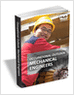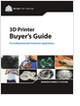Formula HomePlate TheoryClassical PlateKinematicsConstitutiveResultantsEquilibriumSign ConventionPlate CalculatorsCalculator ListMaterial DataElastic ModuliResourcesBibliography
3D Scanners

A white paper to assist in the evaluation of 3D scanning hardware solutions.

Mechanical Engineers Outlook

Guide for those interested in becoming a mechanical engineer. Includes qualifications, pay, and job duties.

CNC Machining Design Guide

Selecting the Right 3D Printer

Discover how to choose the right 3D printer for your needs and the key performance attributes to consider.

more free publicationsForce EquilibriumThe equilibrium equations describe how the plate carries external pressure loads with its internal stresses. To enforce equilibrium, consider the balance of forces and moments acting on a small section of plate. There are six (6) equilibrium equations, three for the forces and three for the moments, that need to be satisfied. The equations of force equilibrium are x direction:y direction:z direction:where Nx, Ny, Nxy, Nyx, Qxz, and Qyz are force resultants; px, py, and pz are distributed external forces applied on the plate. The equations of moment equilibrium are x direction:y direction:z direction:where Mx, My, Mxy, Myx, Nxy, and Nyx are moment resultants; and mx, my, and mz are distributed external moments applied on the plate. Note that all second and higher order terms are neglected.
 Further SimplicificationTo further simplify the problem, consider a plate subjected to transverse loads. In other words, only pz is the non-zero external force. All forces and moments in other directions are zero: px=py=mx=my=mz=0 The above six equations of equilibrium becomeDue to the lack of external force components other than pz, the shear stresses at any given point are paired as follows:This yields3D Scanners

A white paper to assist in the evaluation of 3D scanning hardware solutions.Mechanical Engineers Outlook

Guide for those interested in becoming a mechanical engineer. Includes qualifications, pay, and job duties.CNC Machining Design Guide